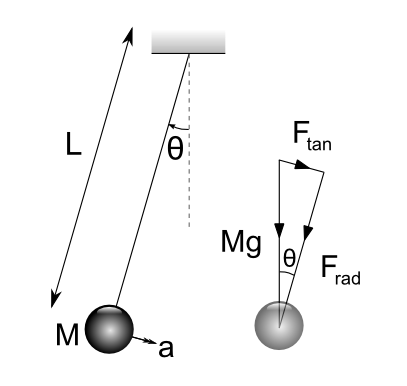#### You may also like### It's Only a Minus Sign

Solve these differential equations to see how a minus sign can change the answer### Differential Equation Matcher

Match the descriptions of physical processes to these differential equations.### Taking Trigonometry Series-ly

Look at the advanced way of viewing sin and cos through their power series.

# The Not-so-simple Pendulum 1

##### Age 16 to 18Challenge Level

We were sent a wonderfully detailed solution by Ben from Kenny. He has formatted it beautifully, so we are including his pdf directly. It is well worth reading through.

Furthermore, one of our vacation summer students produced the solution given here.

### Equation of motionConsidering the forces acting on the pendulum bob acting in the tangential direction
$$F_{tan} = Mg \sin \theta$$
Newton's Second Law:
$$\sum_{i}\mathbf{F_i} = \frac{d}{dt}(m \mathbf{v})$$
Applying tangentially:
$$\Rightarrow M a_{tan} = M g \sin \theta \ \Rightarrow \ a_{tan} = g \sin \theta$$
Changing from translational to angular acceleration $a_{tan} = -\ell \ddot{\theta}$ (negative sign due to opposing directions):
$$\Rightarrow-\ell \ddot{\theta} = g \sin \theta$$
$$\therefore \ddot{\theta} = \frac{-g}{\ell} \sin \theta$$
This is a second-order non-linear differential equation in $\theta$. It cannot be solved easily due to the $\sin \theta$ term. An analytical solution is possible, using what are called elliptical integrals, though it is not reducible to elementary functions.
Comparing with $\ddot{\theta} = -k^2 \sin \theta$ gives
$$k^2 = \frac{g}{\ell} \Rightarrow \ell = \frac{g}{k^2}$$
$$k = 0.1 \Rightarrow k^2 = 0.01 \Rightarrow \ell = 100g = 981\textrm{ m}$$
$$k =1 \Rightarrow k^2 = 1 \Rightarrow \ell = g = 9.81\textrm{ m}$$
$$k =10 \Rightarrow k^2 = 100 \Rightarrow \ell = \frac{g}{100} = 0.0981\textrm{ m}$$

### Linearisation using small-angle approximation

The Taylor series expansion of the sine function is:

$$\sin \theta = \theta - \frac{\theta^3}{3!} + \frac{\theta^5}{5!} - \frac{\theta^7}{7!} + O(\theta^9)$$

(where $O(\theta^7)$ indicates terms in $\theta^9$ and above, sometimes called Big-O notation).

For 'small' $\theta$, $O(\theta^3) \ll \theta$ and so $\sin \theta \approx \theta$. 'Small' here has intentionally been left vague as the next part of the question is to consider the validity of this assumption.

To find the range of values for which $\sin \theta$ lies within 1% of $\theta$, find the point at which $\sin \theta$ is exactly $0.99\theta$ (i.e. 1% less).

$\sin \theta = 0.99 \theta$ by numerical methods $\Rightarrow \theta = \pm 0.24532\ \textrm{rad} = \pm 14.1^\circ$

$|\sin \theta | \leq | \theta |$ for all $\theta$ (with equality only at $\theta = 0$). This can be seen by considering plots of $y=x$ and $y=\sin x$ or using the Taylor series expansion - the sum of the negative terms will always be greater than the sum of the postive terms of order 3 and above.

Therefore $|0.99\theta| \leq |\sin \theta| \leq |\theta|$ for $|\theta| \leq 14.1^\circ$

As $| \theta | \geq |\sin \theta |$, the approximated acceleration will always be greater than the exact value (except for $\theta = 0$ where there will be equality). The period of oscillation of the pendulum will therefore be marginally shorter than in the approximation, i.e. the approximated time period will be too small when compared with (idealised) reality.

Using the $\sin \theta \approx \theta$ approximation, the differential equation becomes:

$$\ddot{\theta} = -k^2\theta$$

This is the equation for simple harmonic motion, with solution of the form:

$$\theta = A\cos(k\theta) + B\sin(k\theta)$$
$$\dot{\theta} = -kA\sin(k\theta) + kB\cos(k\theta)$$

Using the initial conditions $\theta(0) = \theta_0$ and $\dot{\theta}(0) = 0$:

$$\theta_0 = A(1) + B(0) \Rightarrow A = \theta_0$$
$$0 = -kA(0) + kB(1) \Rightarrow B = 0$$

$$\therefore \theta = \theta_0\cos(k\theta)$$

The angular velocity of the (approximated) pendulum is therefore $k$ and so the period is $T = \frac{2\pi}{k}$. The lower bound of the period of a pendulum with length 1m is therefore:

$$k = \sqrt{\frac{g}{\ell}} = \sqrt{g} \Rightarrow T = \frac{2\pi}{k} = \frac{2\pi}{\sqrt{g}}\ \textrm{s}$$